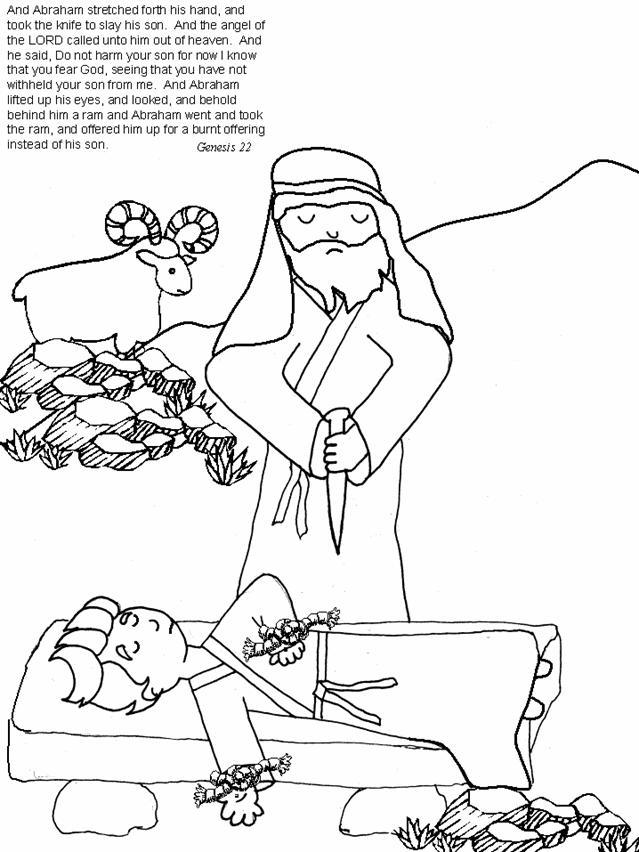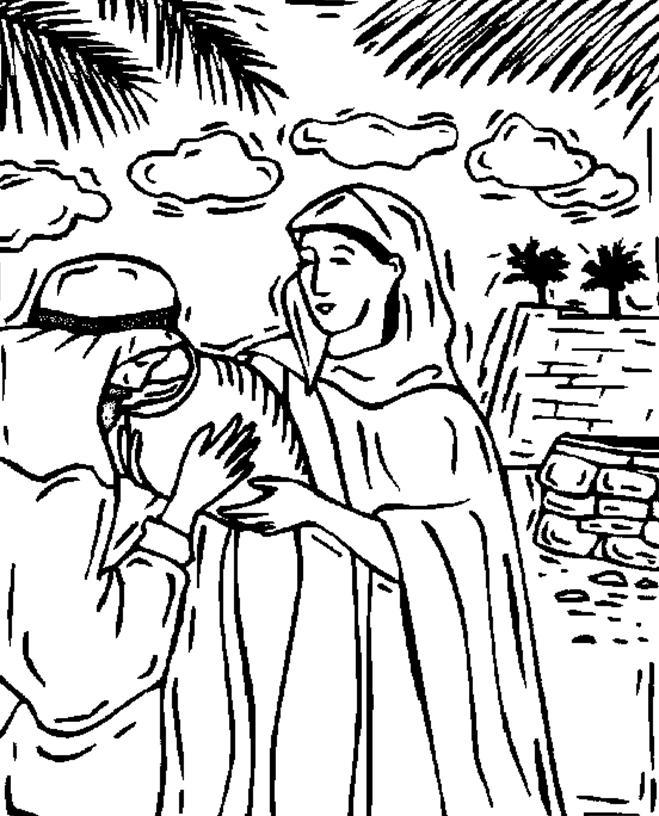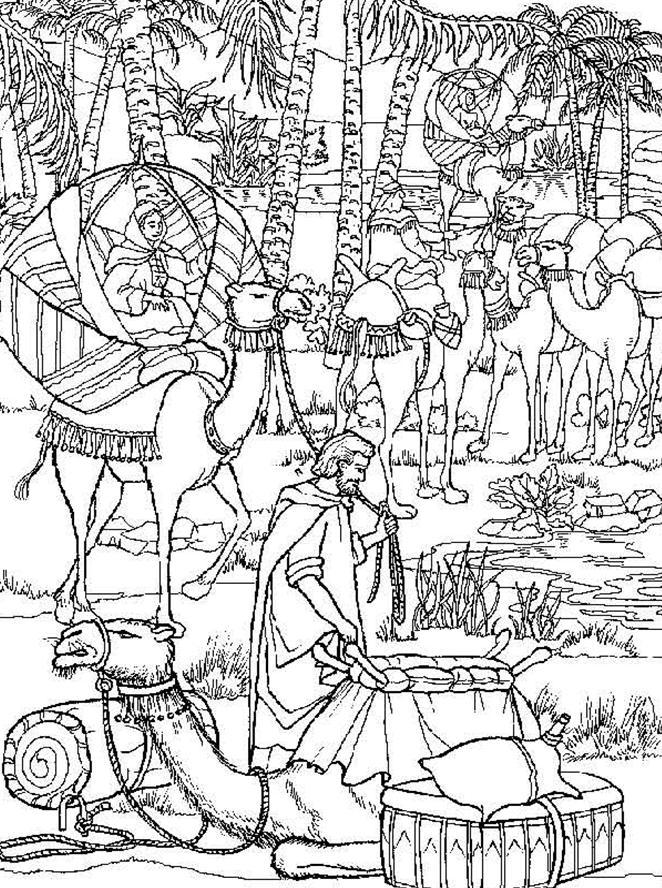# Dr. Pat's Orthodox Super Sunday School Curriculum

## Isaac

ISAAC

Home activities:

• No, you don’t have to visit the zoo again to see the sheep, but it would be fun to go to a petting zoo.
• Look at pictures of babies, maybe even of your own early days. If you know someone
• who’s pregnant, visit and discuss where babies come from.
• How about a jewelry store to look at wedding rings. Compare with Rebekah’s wedding
• jewelry.
• Puzzle and coloring sheet.
• Read the stories of Isaac at bedtime.

Prayer: Lord, thank You that we are wonderfully made, even as the baby Isaac.

# Find these words from the life of Isaac

`M U Z C R Y S H E M W I L C K `
`C A A V E A A W F N H Z E N Z `
`G Q H S R K E H W I C P A R R `
`V E I A E E M E Z N A X M A Y `
`N N H B R R H N N N N V H G J `
`M A E O M B V J I P A M S A A `
`A R B Q E E A A E Y A H I H C `
`R K C A M E L E N B N Y O C O `
`O F X L L B H H E T X O M R B `
`U S O J N S M L H V P C F N J `
`Q R M N N Z H U T L U W A K Y `
`D P I C A E S Y Y K H R J E X `
`K E T U R A H G N I R H Q I Q `
`Q D O Z S V T E L E C A R B U `
`U D D N C A A S I Z P Y U B V `

`ABRAHAM`
`BRACELET`
`CAMEL`
`CANAAN`
`ESAU`
`HAGAR`
`ISAAC`
`ISHMAEL`
`JACOB`
`KETURAH`
`LABAN`
`LORD`
`NAHOR`
`RAM`
`REBEKAH`
`RING`
`SARAH`
`SERVANT`
`SHEEP`

Coloring pages:Rebecca at the WellREBECCA COMES TO ISAAC